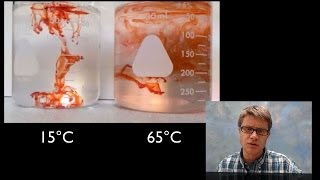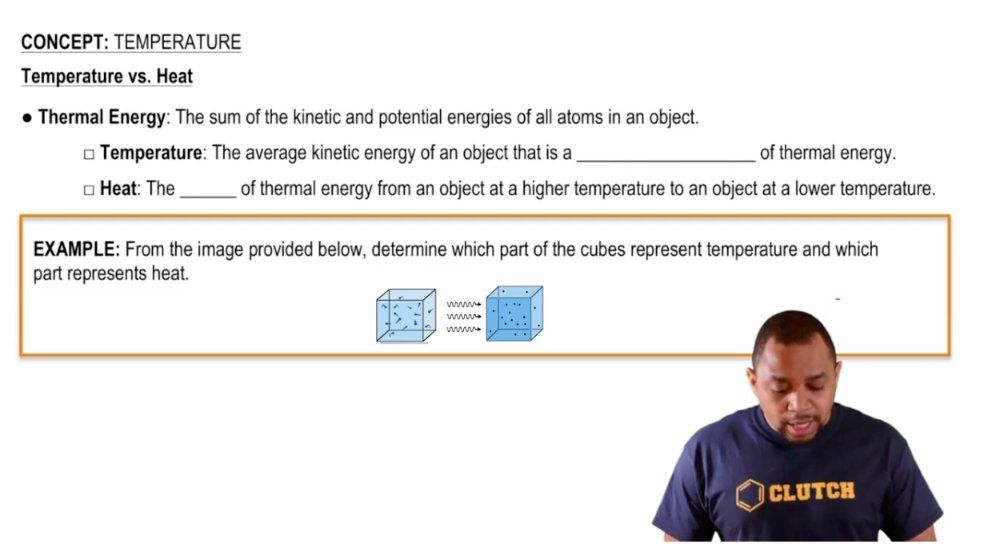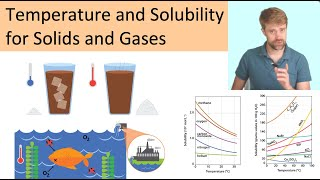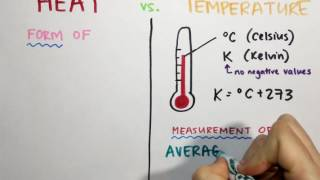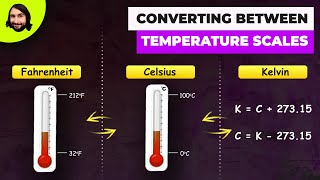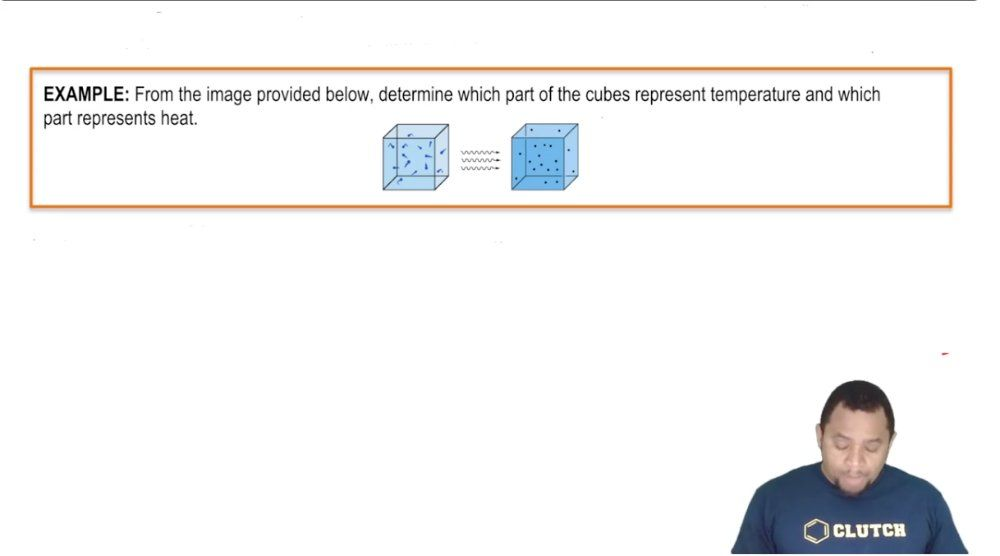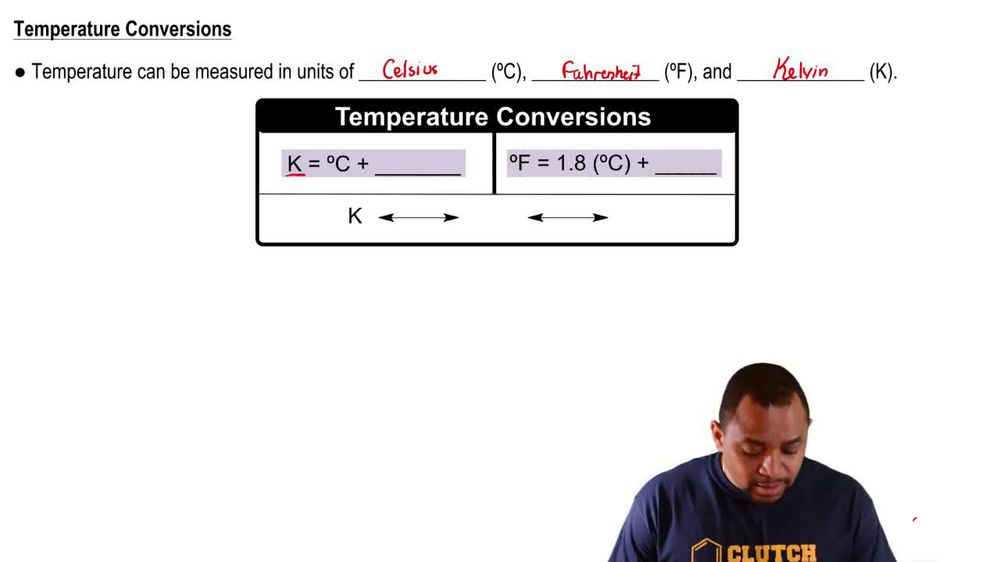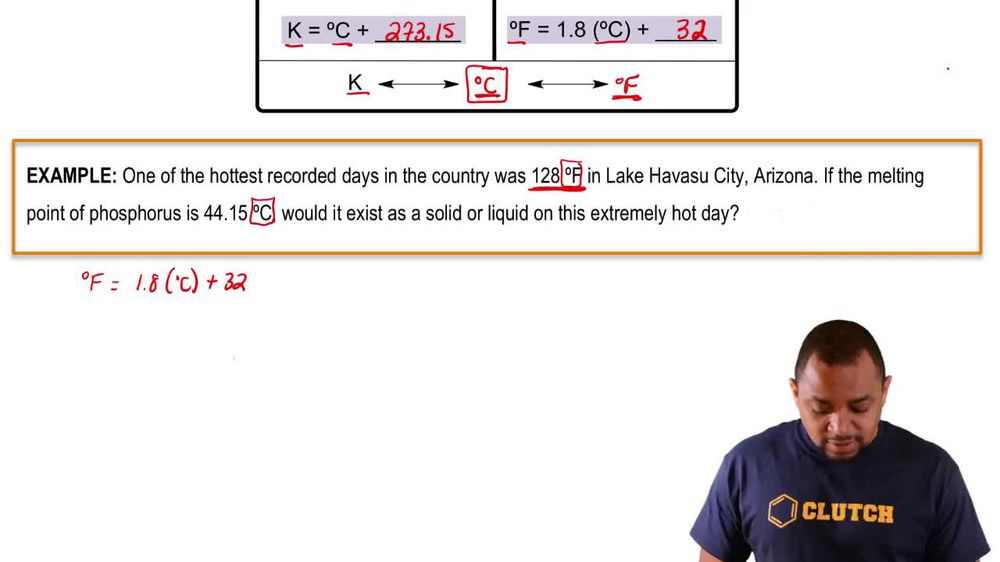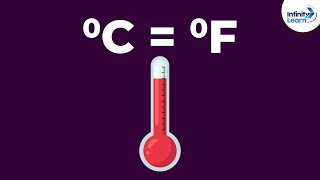Start typing, then use the up and down arrows to select an option from the list.
1. 1. Intro to General Chemistry2. Temperature# Temperature Example 2

by Jules Bruno
149 views
2
0
Let's take a look at the following example question here. It says one of the hottest recorded days in the country was 128 F in Lake Havasu City, Arizona. If the melting point of phosphorus is 44 15 degrees Celsius, what it exists as a solid or liquid on this extremely hot day? Alright, so we see that in the question. We're dealing with units of Fahrenheit and Celsius from the equations up above. We know what's the second one we're gonna have to use in some way. So we have degrees Fahrenheit equals 1.8 times degrees Celsius plus 32. They're giving me the temperature in Lake Havasu in Fahrenheit by me. I need to compare it to this melting point of phosphorus, which is in Celsius. If the temperature I find is equal to or greater than 44. degrees Celsius, that means that phosphorus will melt and it will be in its liquid. For if the temperature on this extremely hot day is not at least 44.15 degrees Celsius, then it won't be hot enough, and phosphorus will not melt and remain a solid so I'm gonna plug in what I have referred. Height, which is 1 28 and this is 1.8 degrees Celsius plus 32 subtract 32 from both sides. So when we do that, we get 96 equals 1.8 times degree Celsius. Divide both sides now by 1.8, and now we'll have the temperature in Lake Havasu in degrees Celsius, so our degrees Celsius here equals 53.3. Now we needed the temperature to would be at least 44.15 degrees Celsius for phosphorus to mount. This answer is much greater than it. So yes, it's hot enough for phosphorus to mount. Therefore, it will exist in its liquid form. Now that we've seen this example on how to relate Fahrenheit two degrees Celsius, move onto the next video and let's take a look at the practice question